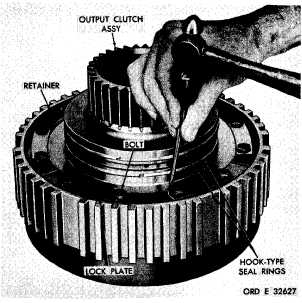Custom SearchPAR  1 6 6 - 1 68 L E F T ,     R I G H T     O U T P U T     C L U T C H E S     R E B U I L D C H A P   5,   SEC  X X I 166.   ASSEMBLY   (figs.   377   and   381, fold-outs   6   and   10) a .    L e f t -    a n d    R i g h t - b r a k e    A n c h or R i n g     A s s e m b l i es .   The   left-   and   right-brake   anchor r i n g    a s s e m b l i e s    a r e    i d e n t i c a l ,    t h e r e - fore    the    following    assembly    procedures apply   to   either   assembly. (1)   Install   the   brake   return   guide   pin   and spring   in   the   brake   anchor   ring   (fig.   207). ( 2 )    U s i n g    a    C - c l a m p ,    c o m p r e s s    t h e brake   return   spring,   and   install   the   retaining ring   (fig.   207). (3)   Install   the   remaining   eight   brake   re- turn   guide   pins,   springs   and   retaining   rings in   the   same   manner   as   described   in   (1)   and   (2), a b o v e. Section 1 6 7 .    D E S C R I P T I ON Refer   to   par.   16   for .   L e f t -    a n d    R i g h t - c l u t c h    R e a c t i on P l a t e    A s s e m b l i e s Note. The   left-   and   right-clutch   re- action   plate   assemblies   are   identical, therefore    the    following    assembly    pro- c e d u r e s    a p p l y    t o    e i t h e r    a s s e m b l y. However,   the   following   describes   the a s s e m b l y    o f    t h e    l e f t - c l u t c h    r e a c t i on p l a t e    a s s e m b l y. ( 1 )    I n s t a l l    t w o    p r e f o r m e d    p a c k i n g s    70 (fig.   377,   fold-out   6)   on   sleeve   71. ( 2 )    I n s t a l l    s l e e v e    7 1    w i t h    p r e f o r m e d packings   70   in   clutch   reaction   plate   67. (3)   Secure   sleeve   71   with   three   5/16-18 x   1   self-locking   bolts   72.   Torque   the   bolts   to 17-20    pound-feet. X X I .    L E F T -    A N D    R I G H T - O U T P U T    C L U T C H E S    —    R E B U I LD the   description   of   the left-   and   right-output   clutches. 168.   DISASSEMBLY   (figs.   377   and   381, fold-outs   6   and   10) .   The   left-   and   right-output   clutch a s s e m b l i e s    a r e    i d e n t i c a l ,    t h e r e f o r e    t he following    disassembly    procedures    apply t o    e i t h e r    a s s e m b l y .    A l l    r e f e r e n c e s    t o exploded   views   are   to   fig.   377,   fold-out 6,   illustrating   the   left   assembly. .   Remove   two   hook-type   seal   rings   from the   hub   of   the   output   clutch   assembly   (fig.   208). .   U s i n g    a    h a m m e r    a n d    p u n c h ,    f l a t t en the   corners   of   the   lock   plates   on   the   output clutch   assembly   (fig.   208). Figure  208.  Straightening  corners  of  output  clutch lock  plates 1 4 3Integrated Publishing, Inc. - A (SDVOSB) Service Disabled Veteran Owned Small Business